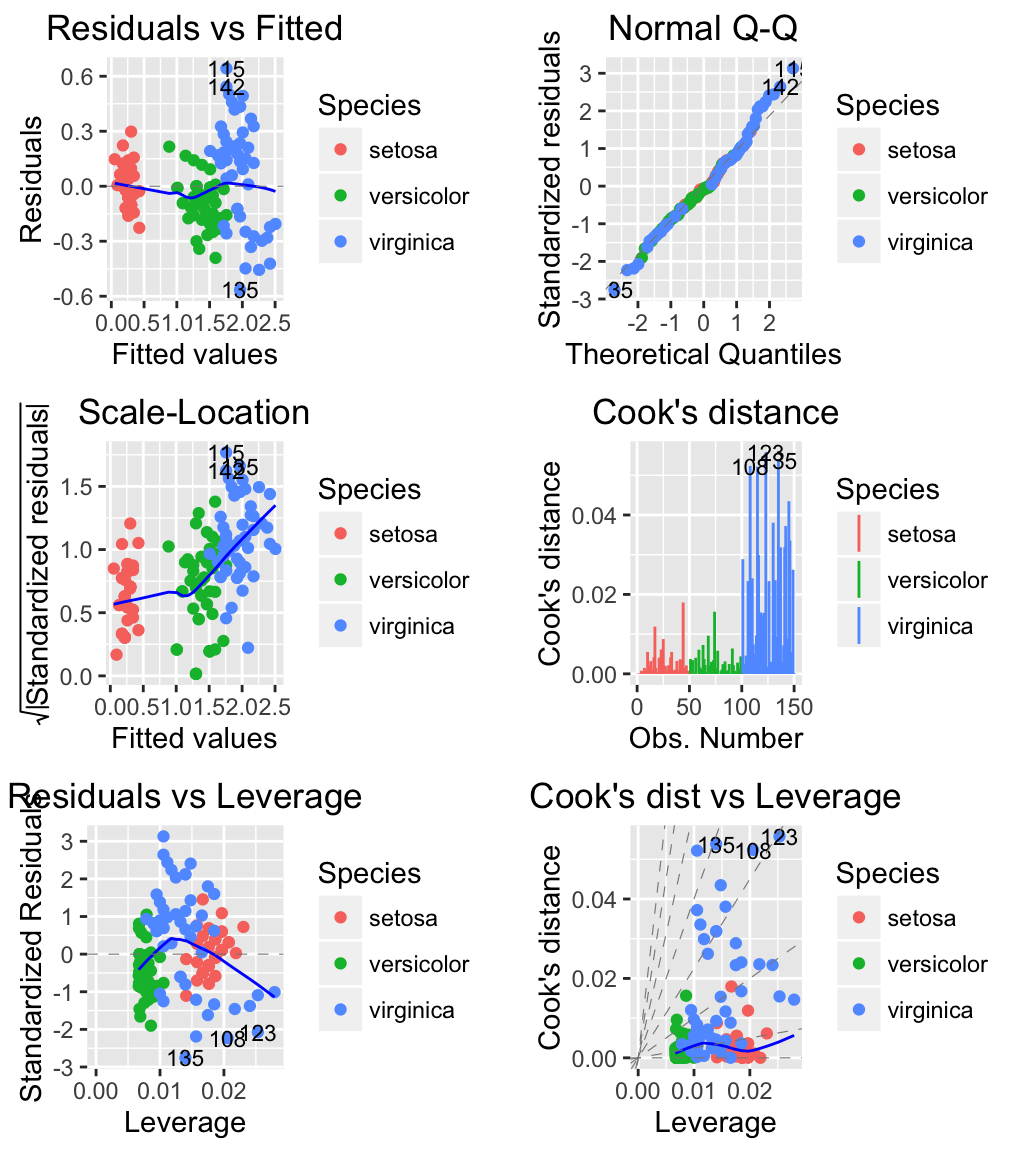# ggfortify : Extension to ggplot2 to handle some popular packages - R software and data visualization

ggfortify extends ggplot2 for plotting some popular R packages using a standardized approach, included in the function autoplot().

• a matrix,
• a scatter plot,
• diagnostic plots for linear model,
• time series,
• the results of principal component analysis,
• the results of clustering analysis,
• and survival curves

The following R packages and functions are covered in the package ggfortify:

Package name Functions
base matrix and table
cluster clara, fanny and pam
changepoint cpt
dlm dlmFilter and dlmSmooth
fGarch fGARCH
forecast bats, forecast, ets and nnetar
fracdiff fracdiff
glmnet glmnet
KFAS KFS and signal
lfda klfda and self
MASS isoMDS and sammon
stats acf, ar, Arima, smdscale, decomposed.ts, density, fractanal, glm, HoltWinters, kmeans, lm, prcomp, princomp, spec, stepfun, stl and ts
survival survfit and survfit.cox
strucchange breakpoints and breakpointsfull
timeSeries timeSeries
tseries irts
vars varprd
xts xts
zoo zooreg

# Installation

ggfortify can be installed from GitHub or CRAN:

``````# Github
if(!require(devtools)) install.packages("devtools")
devtools::install_github("sinhrks/ggfortify")``````
``````# CRAN
install.packages("ggfortify")``````

``library("ggfortify")``

# Plotting matrix

The function autoplot.matrix() is used:

``autoplot(object, geom = "tile")``
• object: an object of class matrix
• geom: allowed values are “tile” (for heatmap) or “point” (for scatter plot)

The mtcars data set is used in the example below.

``````df <- mtcars[, c("mpg", "disp", "hp", "drat", "wt")]
df <- as.matrix(df)``````

Plot a heatmap:

``````# Heatmap
autoplot(scale(df))``````Plot a scatter plot: The data should be a matrix with 2 columns named V1 and V2. The R code below plots mpg by wt. We start by renaming column names.

``````# Extract the data
df2 <- df[, c("wt", "mpg")]
colnames(df2) <- c("V1", "V2")
# Scatter plot
autoplot(df2, geom = 'point') +
labs(x = "mpg", y = "wt")``````# Plotting diagnostics for LM and GLM

The function autoplot.lm() is used to draw diagnostic plots for LM and GLM [in stats package].

``autoplot(object, which = c(1:3, 5))``
• object: stats::lm instance
• which: If a subset of the plots is required, specify a subset of the numbers 1:6.
• ncol and nrow allows you to specify the number of subplot columns and rows.

## Diagnostic plots for Linear Models (LM)

iris data set is used for computing the linear model

``````# Compute a linear model
m <- lm(Petal.Width ~ Petal.Length, data = iris)
# Create the plot
autoplot(m, which = 1:6, ncol = 2, label.size = 3)````````````# Change the color by groups (species)
autoplot(m, which = 1:6, label.size = 3, data = iris,
colour = 'Species')``````## Diagnostic plots with Generalized Linear Models (GLM)

USArrests data set is used.

``````# Compute a generalized linear model
m <- glm(Murder ~ Assault + UrbanPop + Rape,
family = gaussian, data = USArrests)
# Create the plot
# Change the theme and colour
autoplot(m, which = 1:6, ncol = 2, label.size = 3,
colour = "steelblue") + theme_bw()``````# Plotting time series

## Plotting ts objects

• Data set: AirPassengers
• R Function: autoplot.ts()
``autoplot(AirPassengers)``The function autoplot() can handle also other time-series-likes packages, including:

• zoo::zooreg()
• xts::xts()
• timeSeries::timSeries()
• tseries::irts()
• forecast::forecast()
• vars:vars()

## Plotting with changepoint package

The changepoint package provides a simple approach for identifying shifts in mean and/or variance in a time series.

ggfortify supports cpt object in changepoint package.

``````library(changepoint)
autoplot(cpt.meanvar(AirPassengers))``````## Plotting with strucchange package

strucchange is an R package for detecting jumps in data.

Data set: Nile

``````library(strucchange)
autoplot(breakpoints(Nile ~ 1))``````# Plotting PCA (Principal Component Analysis)

• Data set: iris
• Function: autoplot.prcomp()
``````# Prepare the data
df <- iris[, -5]
# Principal component analysis
pca <- prcomp(df, scale. = TRUE)
# Plot
data = iris, colour = 'Species')``````# Plotting K-means

• Data set: USArrests
• Function: autoplot.kmeans()

The original data is required as kmeans object doesn’t store original data. Samples will be colored by groups (clusters).

``````autoplot(kmeans(USArrests, 3), data = USArrests,
label = TRUE, label.size = 3, frame = TRUE)``````# Plotting cluster package

ggfortify supports cluster::clara, cluster::fanny and cluster::pam classes. These functions return object containing original data, so there is no need to pass original data explicitly.

The R code below shows an example for pam() function:

``````library(cluster)
autoplot(pam(iris[-5], 3), frame = TRUE, frame.type = 'norm')``````# Plotting Local Fisher Discriminant Analysis

``````library(lfda)
# Local Fisher Discriminant Analysis (LFDA)
model <- lfda(iris[,-5], iris[, 5], 4, metric="plain")
autoplot(model, data = iris, frame = TRUE, frame.colour = 'Species')``````

# Plotting survival curves

``````library(survival)
fit <- survfit(Surv(time, status) ~ sex, data = lung)
autoplot(fit)``````# Infos

This analysis has been performed using R software (ver. 3.2.1) and ggplot2 (ver. 1.0.1)